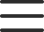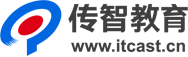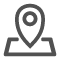# c++培训之基础变量

## 变量

### 不同类型常量的表示

10 整型
10l   10L 长整型
10ll    10LL   长长整型
10ul 10UL 无符号长整型
10ull 10ULL 无符号长长整型
010 八进制8
0x10 十六进制16

### 整型变量的取值范围

|N|为正数且|N| >= 1N的补码为
N的补码 = 2^7 + 2^7 - 1 - |N| + 1 = 2^7 + 2^7 - |N|
M为正数，

2^7 + 2^7 - |N| + M = 2^7 + 2^7 + (M - |N|)
1）如果M > |N|，那么最终运算结果为M - |N|，符号位从1变为0，这是因为N的符号位等于1，可表示为1000 0000 = 2^7，当再加上2^7后，将变为0，即

1000 0000
+   1000 0000

=   10000 0000，1被舍弃了
2）如果M < |N|，那么最终运算结果为2^7 + 2^7 - (|N| - M)，即等于-(|N| - M)的补码，也就是-(|N| - M)了。
3）如果M = |N|，那么最终运算结果为0，溢出位被舍弃了，这与M > |N|的情况类似。
M也为负数的时候，虽然符号位相加，最高位变为0，但由于非符号位相加也存在溢出现象，符号位又变回了1，即负数加上负数还是负数，这是合理的。用公式表示如下，
N的补码 + M的补码 = 2^7 + 2^7 - |N| + 2^7 + 2^7  - |M| = 2^7 + 2^7 - (|N| + |M|)